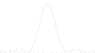# Single slit Diffraction

2Pac
A single slit diffracts laser light of wavelength 610 nm onto a screen 2.25 m away. The distance between the two first-order maxima on either side of the central peak is 4.00 mm. How wide is the slit?

Anyone give me some help? i got an answer of .001029m and apparently this isn't correct. i used the formula for locating the minima $$a\cdot \sin(\theta) = (\lambda)$$ . Since the second minima is located where $$(\lambda)$$ is multiplied by 2. i multiplied the equation on the right side by 3/2 assuming the maxima would be found between both minima.

Staff Emeritus
Gold Member
2Pac said:
Since the second minima is located where $$(\lambda)$$ is multiplied by 2.

•